## Calculus-Integrals

Version 1.0.6 (12 MB) by
Interactive examples using MATLAB to visualize and practice integral calculus and a calculus flashcards app.

Updated Tue, 13 Sep 2022 21:54:13 +0000

From GitHub

# Calculus-Integrals

Curriculum Module
Created with R2021b. Compatible with R2021b and later releases.

## Description

This curriculum module contains interactive live scripts and a MATLAB® app that teach basic concepts of integral calculus. There is a focus on numerical approximation and graphical representation as tools for understanding the concepts of integral calculus.

Calculus-Integrals covers Riemann sum approximations to definite integrals, indefinite integrals as antiderivatives, and the fundamental theorem of calculus. It also covers the indefinite integrals of powers, exponentials, natural logarithms, sines and cosines as well as substitution and integration by parts. Applications include area and power.

These live scripts can be used as part of a lecture, as activities in an instructional setting, or as interactive assignments to be completed outside of class. The module is divided into three folders with the same content offered for different use: Full Scripts, Visualizations, and Practice.

Opening `Integrals.prj` will add the module to your MATLAB path and open a navigation page where you can choose interactive examples by topic or type. The instructions inside the live scripts will guide you through the exercises and activities. Get started with each live script by running it one section at a time. To stop running the script or a section midway (for example, when an animation is in progress), use the Stop button in the RUN section of the Live Editor tab in the MATLAB Toolstrip.

## Prerequisite Domain Knowledge

This module assumes a knowledge of functions that is standard in precalculus course materials regarding powers, exponentials, absolute values, logarithms, sines, cosines, rational functions, and asymptotes. It also assumes knowledge of basic area formulas, including the area of a trapezoid. With the exception of integralRiemann.mlx and vizRiemann.mlx, the scripts are written to follow Calculus-Derivatives and expect basic understanding of derivatives and derivative rules.

## Suggested Prework

Minimal use of MATLAB syntax is required to complete some of the interactive practice, but it is all reviewed in the scripts themselves as needed. If you wish a more complete introduction to MATLAB, you can take MATLAB Onramp – a free two-hour introductory tutorial that teaches the essentials of MATLAB.

Looking for more? Find an issue? Have a suggestion? Have questions?
Contact the MathWorks online teaching team.

# Details

## FullScripts Folder

This folder includes scripts that integrate visualizations with instructional content and practice for active instruction or supported review.

An interactive script that facilitates exploration of simple geometric integral approximations including both rectangular and trapezoidal approximation methods. This script includes an analysis of real power generation data as well as visualizations of the error in each approximation method.

Learning Goals:

• Explain and apply the different approximations computed by a left-endpoint, right-endpoint, midpoint, maximum, or minimum method of selecting a height value in a Riemann sum.
• Discuss the implications for applying calculus in applications with values that are discrete or continuous.
• Explain and apply the trapezoidal approximation.

An interactive script that facilitates exploration of the results of applying the integral as an antiderivative and presents opportunities for practice with immediate feedback.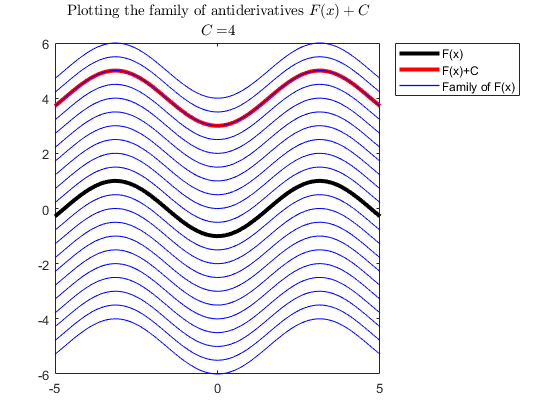Learning Goals:

• Compute integrals of linear combinations of powers, sines, cosines, and exponentials by hand.

An interactive script that presents visualizations of u-substitution and integration by parts as well as scaffolded and randomized practice with immediate feedback.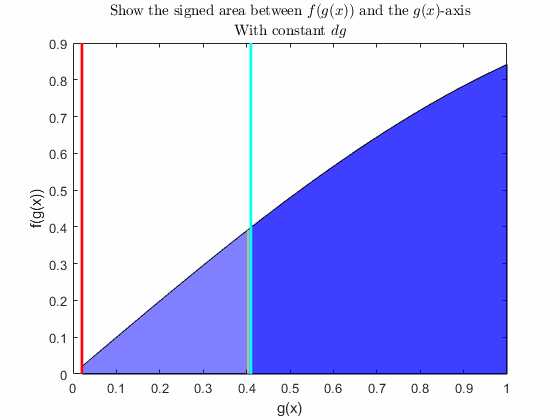Learning Goals:

• Explain what the method of substitution is and how it works.
• Compute integrals of combinations of powers, sines, cosines, exponentials and logarithms that are solvable by substitution by hand.

An interactive script that presents visualizations of integration by parts as well as focused and randomized practice with immediate feedback.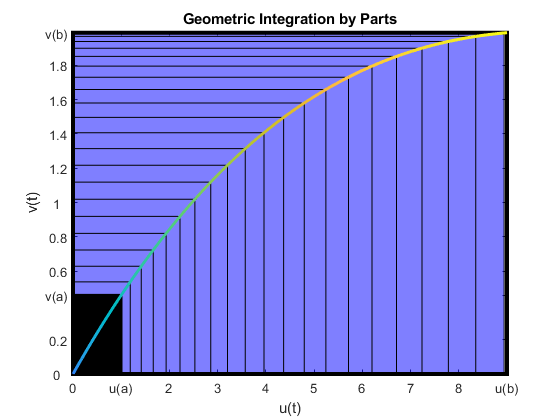Learning Goals:

• Explain what the method of integration by parts is and how it works.
• Compute integrals involving powers, sines, cosines, exponentials and logarithms that are solvable by integration by parts by hand.

An interactive script that presents visualizations of the fundamental theorem of calculus as well as an opportunity to practice computing definite integrals by hand with immediate feedback.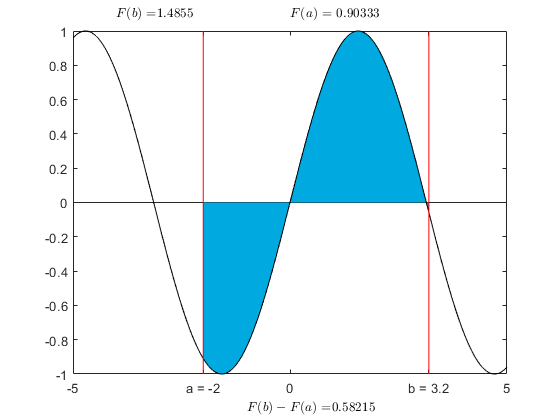Learning Goals:

• Explain the fundamental theorem of calculus.
• Fluently recognize and apply the rules for computing both definite and indefinite integrals.

## Visualizations Folder

This folder includes scripts for the visualization examples alone, as you might use them in a lecture or class demonstration.

An interactive script presenting visualizations of approximating the area under a user-defined curve by a user-defined number of rectangular or trapezoidal components as well as options to picture the error in each approximation. The only addition to this set of examples is the option to use a randomly selected point in each interval to determine the height of the rectangular approximation. This only makes sense in the context of an area defined by a function rather than by data, but could be useful for discussing why we generally just stick with interval endpoints.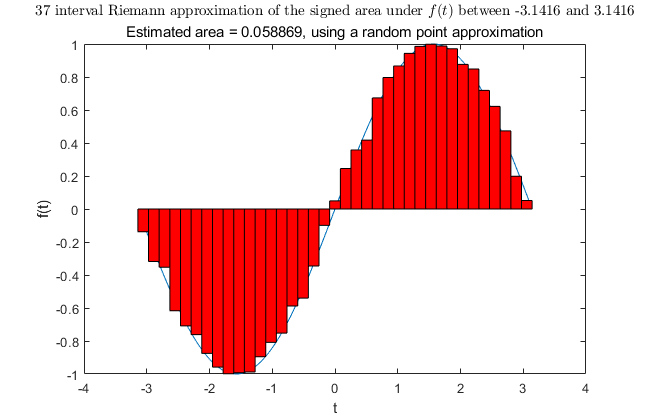Learning Goals:

• Be able to sketch the graphical interpretation of geometric approximations to areas defined by curves and be able to explain why particular methods and particular curves result in especially good (or bad) approximations.
• Explain why increasing the number of intervals in an approximation will decrease the error.

An interactive script presenting geometric interpretations of both the integral and derivative forms of the Fundamental Theorem of Calculus, applied to user-defined functions.Learning Goals:

• Be able to explain why the Fundamental Theorem of Calculus makes sense graphically.

An interactive script presenting visualizations displaying the different curves generated by plotting f(g(x)) against g(x) or against x, as well as exploring the impact of using a step of dx or a step of dg = g'(x)dx to traverse the region of interest. The animation code geometrically demonstrates some of the potential savings by showing a default example where the dx integral adds and subtracts a region that the dg integral entirely ignores.Learning Goals:

• Graphical understanding of the method of substitution.

An interactive script presenting a geometric understanding of integration by parts. The default values generate a classic visualization of a definite integration by parts, but the code is designed to allow investigation of more complicated functions as well by allowing the separation of the resulting image into positive and negative regions with respect to each variable as well as showing or hiding the lines depicting the standard intervals for each variable.Learning Goals:

• Graphical understanding of the integration by parts formula.

An interactive script presenting a visualization of the family of antiderivatives.Learning Goals:

• Graphical understanding of the concept of general antiderivatives.

## Practice Folder

This folder includes scripts that are purely directed at student practice, as you might assign for homework or use for understanding checks during class or offer to students to help them study for an exam.

A MATLAB app that allows the user to select among four different categories of derivative practice (simple derivatives, linear combinations of powers, product rule, and chain rule) or integral practice (simple integrals, definite integrals, substitution, and integration by parts) or any combination thereof. Variables can be restricted to x and t or varied randomly over a larger set of options. The solutions can be displayed immediately or multiple attempts at each problem can be recorded. Results are tracked by type of problem including correct solutions, incorrect solutions, and problems which are not even attempted before generating a new one. Some constructive feedback for common errors is automatically generated.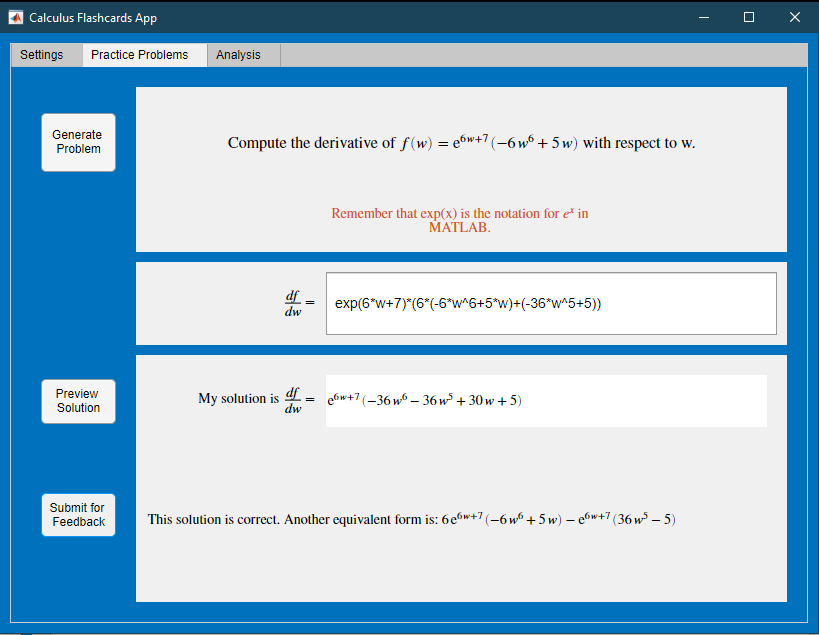Learning Goals:

• Computational fluency with common functions and interactions including linearity, the product rule, and the chain rule for derivatives and linearity, substitution, integration by parts, and the fundamental theorem of calculus for integrals.

An interactive set of randomly generated practice problems on definite integrals, including divergent integrals with finite bounds. The overall success rate within each session is available upon request but is not saved.

Learning Goals:

• Computational fluency for definite integrals involving linear and rational combinations of powers, sines, cosines, exponentials and natural logarithms.

This script pulls together the interactive exercises for each simple antiderivative from `integralAntiderivatives.mlx` as well as the randomized practice.

Learning Goals:

• Computational fluency with the antiderivatives of powers, sines, cosines, and exponentials.

This script pulls together the interactive exercises for substitution from `integralSubstitution.mlx` as well as the randomized practice.

Learning Goals:

• Computational fluency with the application of substitution as a method for solving simple integrals.

This script pulls together the interactive exercises for applying the method of integration by parts presented in `integralByParts.mlx` as well as the randomized practice.

Learning Goals:

• Computational fluency with the application of the method of integration by parts to standard shapes of problems.

## Setup To Use the Calculus Flashcards App

### MATLAB®

1. Ensure that you have MATLAB R2021a or newer installed.
2. Download `CalculusFlashcards.mlapp` or download and unzip the entire repository.
3. Right-click the app in MATLAB and select run or double click on the live script (.mlx file) that you wish to run.

### MATLAB Online™

1. Download `CalculusFlashcards.mlapp` or download and unzip the entire repository.
2. Drag and drop `CalculusFlashcards.mlapp` into the Current Folder in MATLAB Online.
3. Right-click the `CalculusFlashcards.mlapp` and select run or double click on the live script (.mlx file) that you wish to run.

## Products

MATLAB®, Symbolic Math Toolbox™

The license for this module is available in the LICENSE.md file in this GitHub repository.

## Educator Resources

Have any questions or feedback? Contact the MathWorks online teaching team.

### Cite As

Emma Smith Zbarsky (2023). Calculus-Integrals (https://github.com/MathWorks-Teaching-Resources/Calculus-Integrals/releases/tag/v1.0.6), GitHub. Retrieved .

##### MATLAB Release Compatibility
Created with R2021b
Compatible with R2021b and later releases
##### Platform Compatibility
Windows macOS Linux
##### Communities
More Files in the  Distance Learning Community

### Community Treasure Hunt

Find the treasures in MATLAB Central and discover how the community can help you!

Start Hunting!

#### Visualizations

Version Published Release Notes
1.0.6

See release notes for this release on GitHub: https://github.com/MathWorks-Teaching-Resources/Calculus-Integrals/releases/tag/v1.0.6

1.0.5

See release notes for this release on GitHub: https://github.com/MathWorks-Teaching-Resources/Calculus-Integrals/releases/tag/v1.0.5

1.0.4

See release notes for this release on GitHub: https://github.com/MathWorks-Teaching-Resources/Calculus-Integrals/releases/tag/v1.0.4

1.0.3

See release notes for this release on GitHub: https://github.com/MathWorks-Teaching-Resources/Calculus-Integrals/releases/tag/v1.0.3

1.0.2

See release notes for this release on GitHub: https://github.com/MathWorks-Teaching-Resources/Calculus-Integrals/releases/tag/v1.0.2

1.0.0

To view or report issues in this GitHub add-on, visit the GitHub Repository.
To view or report issues in this GitHub add-on, visit the GitHub Repository.